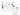# Example: Quantum TeleportationThe quantum circuit uses three qubits and two classical bits. There are four main components in this quantum circuit.

Initialization and reset First, we need to start our quantum computation with a well-defined quantum state. This is achieved using the initialization and reset operations. The resets can be performed by a combination of single-qubit gates and concurrent real-time classical computation that monitors whether we have successfully created the desired state through measurements. The initialization of into a desired state can then follow by applying single-qubit gates.

Quantum gates Second, we apply a sequence of quantum gates that manipulate the three qubits as required by the teleportation algorithm. In this case, we only need to apply single-qubit Hadamard () and two-qubit Controlled-X () gates.

Measurements Third, we measure two of the three qubits. A classical computer interprets the measurements of each qubit as classical outcomes (0 and 1) and stores them in the two classical bits.

Classically conditioned quantum gates Fourth, we apply single-qubit and quantum gates on the third qubit. These gates are conditioned on the results of the measurements that are stored in the two classical bits. In this case, we are using the results of the classical computation concurrently in real-time within the same quantum circuit.

XPERIMEN.COM